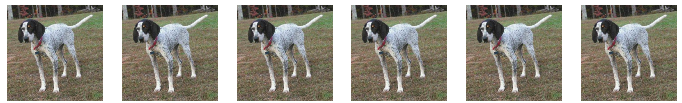# Data Augmentation plots are all the same

I am trying to replicate the “Dog Breed” notebook and I want to take a look at my augmented images. To do so I use the code:

``````def get_data(sz, bs):
tfms = tfms_from_model(arch, sz, aug_tfms=transforms_side_on, max_zoom=1.1)
data = ImageClassifierData.from_csv(wd, 'train', f'{input_path}labels.csv', test_name='test',
val_idxs=val_idxs, suffix='.jpg', tfms=tfms, bs=bs)

return data if sz > 300 else data.resize(340, wd)

data = get_data(299, bs)

x,_ = next(iter(data.aug_dl))